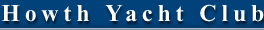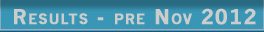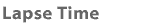Occasionally I'm asked to explain how the "Lapse" time column in a race result is calculated so, here goes! Lapse Time is the True Lapse Time on Winner. It is the time that a boat would need to shave-off its elapsed time in order to have the same corrected time as that of the winning boat in a race result. Lets look at the following result for 2 boats, Boat A, the winner and Boat B. The calculation and reasoning remains the same for any further boats.

 Plc Boat IRCTCF Time ofFinish ElaspedTime CorrectedTime TrueLapse onWinner PointsthisRace 1 Boat A 0.908 11:56:51 1:46:51 1:37:02 1.00 2 Boat B 0.943 12:21:25 2:11:25 2:03:56 28:32 2.00 3 etc

 The calculation is as follows .... For "Boat A" elapsed time    =    1:46:51    =    106.85 min corrected time    =    0.908 ( 106.85 )    =    97.02 min ( which is the 1:37:02 above) For "Boat B" elapsed time    =    2:11:25    =    131.42 min corrected time    =    0.943 ( 131.42 )    =    123.93 min ( which is the 2:03:56 above)

TLT is "True Lapse Time on Winner" below ...

 (Boat B TCF)(TLT) = Boat B Corrected Time - Boat A Corrected Time (0.943)(TLT) = 123.93 - 97.02 TLT = 26.91 / 0.943 TLT = 28.547 min (which is the 28:32 min:sec above)

 So, "Boat B" needs to SHAVE OFF 28:32 min:sec (28.547 min) from its elapsed time in order to have the same CORRECTED TIME as the WINNER ("Boat A"). This definition is fine if one is interested in the time to shave off one's elapsed time to win a race, but I find that most competitors are primarily interested in the simple elapsed time differences between themselves and other boats.